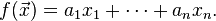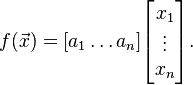# Linear operator, linear functional difference?

What is a difference between linear operator and linear functional?

Do I understand it correctly that linear operator is any operator that when applied on a vector from a vector space, gives again a vector from this vector space. And also obeys linearity conditions.

And linear functional is a vector, dot product of which with the vector from the vector space gives again a vector from this vector space. And also linearity conditions are met.

So, linear functional is a vector and linear operator is not a vector?

ShayanJ
Gold Member
Let V be a vector space defined on a field F(don't worry about the word "field", just think about real numbers). A linear operator is a linear map from V to V. But a linear functional is a linear map from V to F. So linear functionals are not vectors. In fact they form a vector space called the dual space to V which is denoted by $V^*$. But when we define a bilinear form on the vector space, we can use it to associate a vector with a functional because for a vector v, $\langle v, () \rangle: V \rightarrow F$ is a functional in the dual space.

Last edited:
•Goodver
Shyan,
Suppose we have a vector space V(x1,x2,x3) where x1,x2,x3 ∈ R
And a vector u = <1, 2, 3>

Then for instance:

Linear operator: D(u) = 2 * u , u ∈ V
Linear functional: D(u) = <1, 1, 1> ⋅ u , u ∈ V

?

ShayanJ
Gold Member
Shyan,
Suppose we have a vector space V(x1,x2,x3) where x1,x2,x3 ∈ R
And a vector u = <1, 2, 3>

Then for instance:

Linear operator: D(u) = 2 * u , u ∈ V
Linear functional: D(u) = <1, 1, 1> ⋅ u , u ∈ V

?
Yeah, that's right!
But you should note that functionals don't have to be defined in terms of vectors. What I explained is a way of making a correspondence(which technically is called isomorphism) between vectors and functionals.
I can define a functional as $\omega(u)=u_1+u_2+u_3$ which, by your construction, is associated with the vector <1,1,1>, because we have $\omega(u)=D(u)$.
I also recommend you to read the pages that PeroK gave the links to. Because I just answered the question while there are more things to it!

•Goodver
Thank you,

Is it by definition that the inner product of functional with the vector from V has to be a value from the field of the vector space (in our case real number)?

Or these can also be considered as functional for the space V, since they outcome a real number which is an instance from the filed of V?

1. ω(u) = u1
2. ω(u) = u2 + 5
3. ω(u) = 0

ShayanJ
Gold Member
Is it by definition that the inner product of functional with the vector from V has to be a value from the field of the vector space (in our case real number)?
You take the inner product of two vectors, not a functional and a vector(See the link bilinear form above!).
Yes, its the definition of inner product to take two vectors and give a scalar(a member of the field)!
Goodver said:
Or these can also be considered as functional for the space V, since they outcome a real number which is an instance from the filed of V?

1. ω(u) = u1
2. ω(u) = u2 + 5
3. ω(u) = 0
These are functionals too.
I don't see any connection between your two questions that can make you use that "Or" between them!

As far as I understand.

By definition, dual space for vector space V is a vector space consisting of all linear functionals for vector space V.

If a vector space given by components x1,x2 ... xn, then linear functional can be written as a functionOr it can be written in a matrix form:From here, we have a vector space of row vectors [a1 ... an] which form a dual space. (Am I correct?)
Which implies that number of vector dimensions n must be the same for dual vector space and actual vector space.

If n = 3, then

In this case:

1. ω(u) = u1 + u2 + u3

We have <1, 1, 1> vector in a dual vector space, right?

But what are the vectors in the dual vector space formed by these functionals then?

1. ω(u) = u1
2. ω(u) = u2 + 5
3. ω(u) = 0

Last edited:
ShayanJ
Gold Member
From here, we have a vector space of row vectors [a1 ... an] which form a dual space. (Am I correct?)
Yeah, that's correct.
1. ω(u) = u1 + u2 + u3

We have <1, 1, 1> vector in a dual vector space, right?
Yes, this is correct too. But not completely. As I said, you should read some detailed text.

But what are the vectors in the dual vector space formed by these functionals then?

1. ω(u) = u1
2. ω(u) = u2 + 5
3. ω(u) = 0
$1. (1 \ \ 0 \ \ 0) \\ 3. (0 \ \ 0 \ \ 0)$
The second one is not a linear functional:
$\omega(u+v)=(u+v)_1+5=u_1+v_1+5 \neq u_1+5+v_1+5=\omega(u)+\omega(v)$

•Goodver